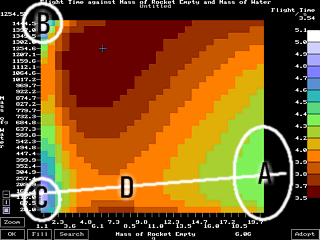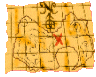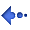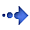Water Rocket Computer Model ProblemsI asked for an explanation for the following . . . 1. to find an explanation for the maximum at the top left (B); and, 2. the maximum at the lower left (C).

Line D is the line that corresponds to the maximum flight time for a given mass of rocket which matches well the maximum height - flight time being related to the amount of time it takes a rocket to fall from the sky.

Area A is the useful maximum for this as it is normally not possible to get a rocket that weighs 1.1 grammes with a capacity of 2 litres.

The far left of the plot has the property that the size of the rocket and its weight is such that the rocket will float down to earth rather than plummet in the usual manner. In this way, if the rocket can be given some altilude by pushing it there, it will take a while to float back to earth. Area B represents a flight where the rocket is pushed up almmost to its maximum altitude by the water at which point it virtually stops and then floats back to earth.

Area C is where this left hand side phenomenon coincides with the maximum height to give another peak.Back to the Index Copyright 1994 - 2003 P.A.Grosse. All Rights Reserved